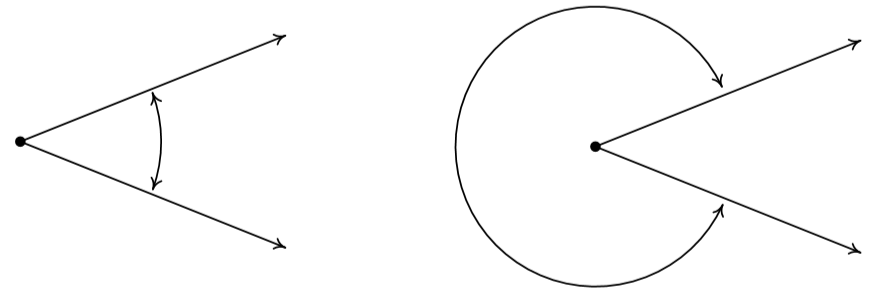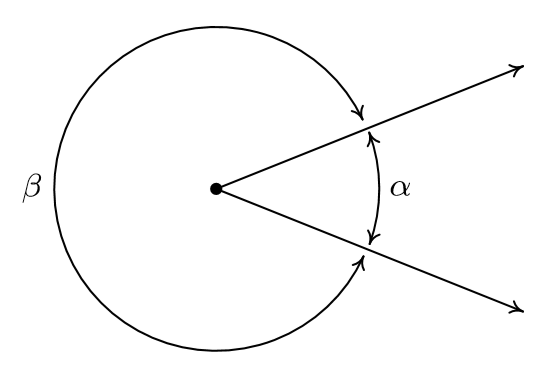$$\newcommand{\id}{\mathrm{id}}$$ $$\newcommand{\Span}{\mathrm{span}}$$ $$\newcommand{\kernel}{\mathrm{null}\,}$$ $$\newcommand{\range}{\mathrm{range}\,}$$ $$\newcommand{\RealPart}{\mathrm{Re}}$$ $$\newcommand{\ImaginaryPart}{\mathrm{Im}}$$ $$\newcommand{\Argument}{\mathrm{Arg}}$$ $$\newcommand{\norm}{\| #1 \|}$$ $$\newcommand{\inner}{\langle #1, #2 \rangle}$$ $$\newcommand{\Span}{\mathrm{span}}$$

# 10.1: Angles and their Measure

$$\newcommand{\vecs}{\overset { \rightharpoonup} {\mathbf{#1}} }$$

$$\newcommand{\vecd}{\overset{-\!-\!\rightharpoonup}{\vphantom{a}\smash {#1}}}$$

This section begins our study of Trigonometry and to get started, we recall some basic definitions from Geometry. A ray is usually described as a half-line' and can be thought of as a line segment in which one of the two endpoints is pushed off infinitely distant from the other, as pictured below. The point from which the ray originates is called the initial point of the ray.A ray with initial point P

When two rays share a common initial point they form an angle and the common initial point is called the vertex of the angle. Two examples of what are commonly thought of as angles are(left) An angle with vertex P. (right) An angle with vertex Q.

However, the two figures below also depict angles - albeit these are, in some sense, extreme cases. In the first case, the two rays are directly opposite each other forming what is known as a straight angle; in the second, the rays are identical so the angle' is indistinguishable from the ray itself.A straight angle.

The measure of an angle is a number which indicates the amount of rotation that separates the rays of the angle. There is one immediate problem with this, as pictured below.Which amount of rotation are we attempting to quantify? What we have just discovered is that we have at least two angles described by this diagram.\footnote{The phrase at least' will be justified in short order.} Clearly these two angles have different measures because one appears to represent a larger rotation than the other, so we must label them differently. In this book, we use lower case Greek letters such as $$\alpha$$ (alpha), $$\beta$$ (beta), $$\gamma$$ (gamma) and $$\theta$$ (theta) to label angles. So, for instance, we haveOne commonly used system to measure angles is \index{angle ! degree}\index{degree measure}\textbf{degree measure}. Quantities measured in degrees are denoted by the familiar $^{\circ}$' symbol. One complete revolution as shown below is $$360^{\circ}$$, and parts of a revolution are measured proportionately.

Note

The odd choice of $360$' is most often attributed to the Babylonians.

Thus half of a revolution (a straight angle) measures $$\frac{1}{2} \left(360^{\circ}\right) = 180^{\circ}$$, a quarter of a revolution (a right angle) measures $$\frac{1}{4} \left(360^{\circ}\right) = 90^{\circ}$$ and so on.Note that in the above figure, we have used the small square $\! \! \! \! \! \! \qed$' to denote a right angle, as is commonplace in Geometry. Recall that if an angle measures strictly between $$0^{\circ}$$ and $$90^{\circ}$$ it is called an \index{acute angle}\index{angle ! acute}\textbf{acute angle} and if it measures strictly between $$90^{\circ}$$ and $$180^{\circ}$$ it is called an \index{obtuse angle}\index{angle ! obtuse}\textbf{obtuse angle}. It is important to note that, theoretically, we can know the measure of any angle as long as we know the proportion it represents of entire revolution (this is how a protractor is graded). For instance, the measure of an angle which represents a rotation of $$\frac{2}{3}$$ of a revolution would measure $$\frac{2}{3} \left(360^{\circ}\right) = 240^{\circ}$$, the measure of an angle which constitutes only $$\frac{1}{12}$$ of a revolution measures $$\frac{1}{12} \left(360^{\circ}\right) = 30^{\circ}$$ and an angle which indicates no rotation at all is measured as $$0^{\circ}$$.Using our definition of degree measure, we have that $$1^{\circ}$$ represents the measure of an angle which constitutes $$\frac{1}{360}$$ of a revolution. Even though it may be hard to draw, it is nonetheless not difficult to imagine an angle with measure smaller than $$1^{\circ}$$. There are two ways to subdivide degrees. The first, and most familiar, is decimal degrees. For example, an angle with a measure of $$30.5^{\circ}$$ would represent a rotation halfway between $$30^{\circ}$$ and $$31^{\circ}$$, or equivalently, $$\frac{30.5}{360} = \frac{61}{720}$$ of a full rotation. This can be taken to the limit using Calculus so that measures like $$\sqrt{2}^{\, \circ}$$ make sense.\footnote{Awesome math pun aside, this is the same idea behind defining irrational exponents in Section \ref{IntroExpLogs}.}

The second way to divide degrees is the Degree - Minute - Second (DMS) system. In this system, one degree is divided equally into sixty minutes, and in turn, each minute is divided equally into sixty seconds. Does this kind of system seem familiar? In symbols, we write $$1^{\circ} = 60'$$ and $$1' = 60''$$, from which it follows that $$1^{\circ} = 3600''$$. To convert a measure of $$42.125^{\circ}$$ to the DMS system, we start by noting that $$42.125^{\circ} = 42^{\circ} + 0.125^{\circ}$$. Converting the partial amount of degrees to minutes, we find $$0.125^{\circ} \left( \frac{60'}{1^{\circ}} \right) = 7.5' = 7' + 0.5'$$. Converting the partial amount of minutes to seconds gives $$0.5' \left(\frac{60''}{1'} \right) = 30''$$. Putting it all together yields

$\begin{array}{rcl} 42.125^{\circ} & = & 42^{\circ} + 0.125^{\circ} \\ & = & 42^{\circ} + 7.5' \\ & = & 42^{\circ} + 7' + 0.5' \\ & = & 42^{\circ} + 7' + 30'' \\ & = & 42^{\circ} 7' 30'' \\ \end{array}$

On the other hand, to convert $$117^{\circ}15'45''$$ to decimal degrees, we first compute $$15' \left(\frac{1^{\circ}}{60'}\right) = \frac{1}{4}^{\circ}$$ and $$45'' \left(\frac{1^{\circ}}{3600''}\right) = \frac{1}{80}^{\circ}$$. Then we find

$\begin{array}{rcl} 117^{\circ}15'45'' & = & 117^{\circ} + 15' + 45'' \\ [5pt] & = & 117^{\circ} + \frac{1}{4}^{\circ} + \frac{1}{80}^{\circ} \\ [5pt] & = & \frac{9381}{80}^{\circ} \\ [5pt] & = & 117.2625^{\circ} \\ \end{array}$

Recall that two acute angles are called complementary angles if their measures add to $$90^{\circ}$$. Two angles, either a pair of right angles or one acute angle and one obtuse angle, are called supplementary angles if their measures add to $$180^{\circ}$$. In the diagram below, the angles $$\alpha$$ and $$\beta$$ are supplementary angles while the pair $$\gamma$$ and $$\theta$$ are complementary angles.## Contributors

• Carl Stitz, Ph.D. (Lakeland Community College) and Jeff Zeager, Ph.D. (Lorain County Community College)